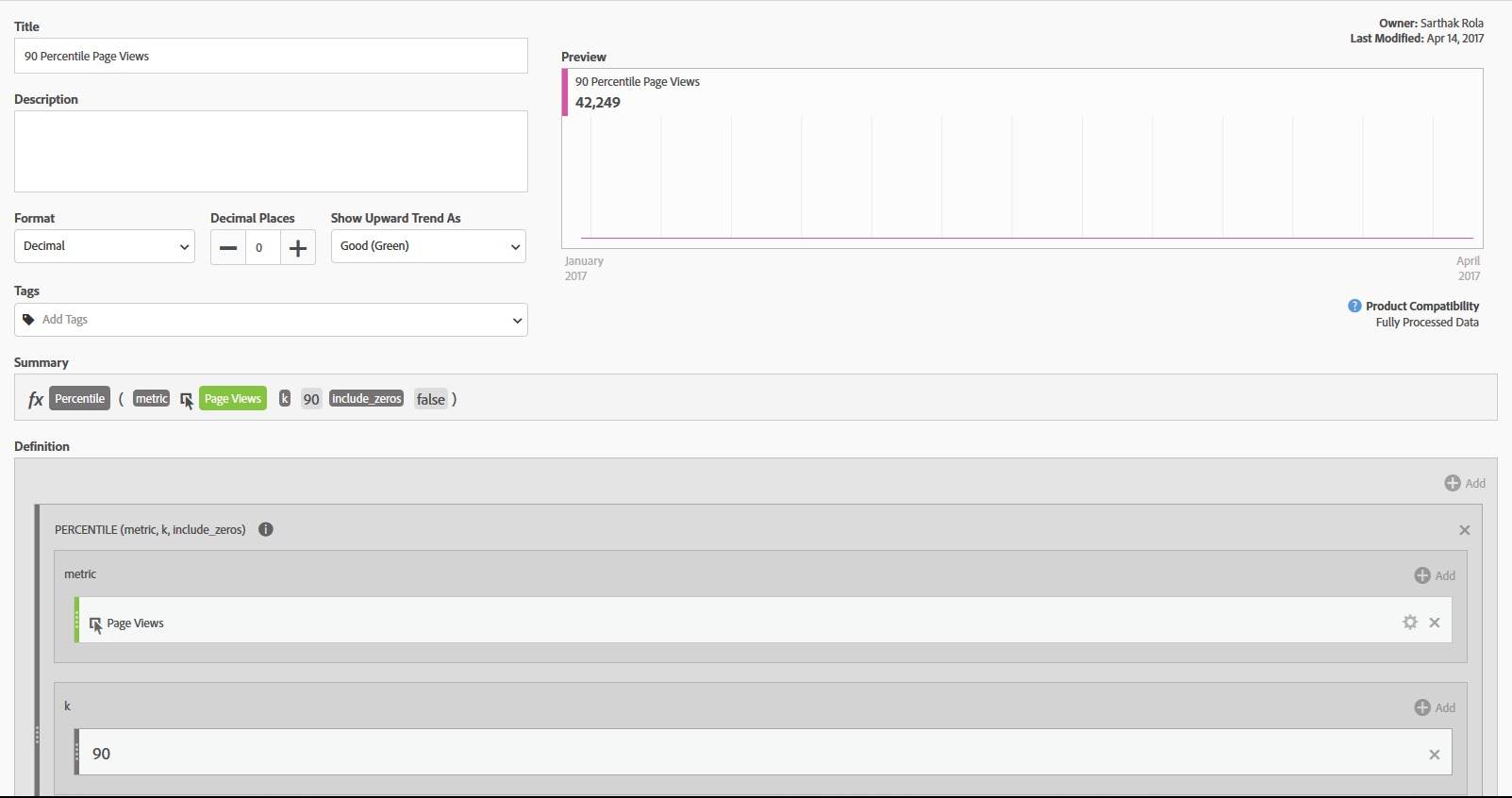For a selected metric, the Percentile function returns the percentile of the data based on the data value (line item and its corresponding metric data) which gets selected based on the Percentile calculation.

The logic for calculating the Percentile is :

Data Value i = (Percentile/100) x (Number of line items +1)

where Data Value "i"= ith line item in the report

Lets consider an example. We want to calculate the 90th percentile for Page Views for Pages Report where we have 422 pages in total. Here is the screen shot of the calculated metric:To calculate it, first the report will be sorted by Page Views in incremental order. As per the equation above, here is the calculation:
(90/100) x (422+1) = 0.8 x 423 = 380.7

Now, Integer Part = 380 and Factorial Part = 0.7

Since the data value is not a whole number, it will be calculated as:

Line Item 380 Data Value +(Factorial Part x (Line Item 381 Data Value - Line Item 380 Data Value)) = Corresponding Percentile value.

Note:

The Percentile function does not work on individual line item basis. It works on an aggregated line item basis hence the value returned in the Calculated Metric will be the same for all of the line items.